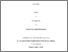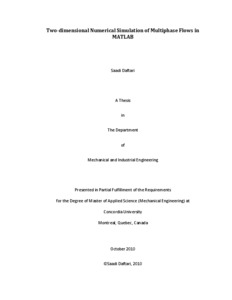Title:

# Two-dimensional Numerical Simulation of Multiphase Flows in MATLAB

Daftari, Saadi (2010) Two-dimensional Numerical Simulation of Multiphase Flows in MATLAB. Masters thesis, Concordia University.Preview Text (application/pdf) DAFTARI_MASc_F2010.pdf - Accepted Version Available under License Spectrum Terms of Access. 3MB

## Abstract

A robust and efficient numerical method for the simulation of incompressible, immiscible, two-phase flows in two dimensions is presented. Following a comprehensive literature review, the one-fluid model is selected to account for the discontinuities in material properties across the interface. The model uses the volume-of-fluid (VOF) method and the continuum surface force (CSF) model to track the interface and account for the surface tension forces, respectively. Integration in time is accomplished explicitly through the forward Euler method and the Navier-Stokes equations are discretized in space using a second-order upwind biased oscillation free Total Variation Diminishing (TVD) method for the convective terms and a second-order central differencing method for the viscous terms. The velocity-pressure coupling is done using a two step first-order projection method. The interface is reconstructed geometrically using a piecewise linear interface calculation (PLIC) method and the VOF advection equation is advanced in time using a directional split algorithm. The final numerical algorithm is implemented in MATLAB where the Pressure Poisson Equation (PPE) obtained from the projection method is solved efficiently in a cluster environment using a built-in MATLAB solver. Various benchmark tests are used to validate the algorithm and a grid refinement test is performed to determine its formal order of accuracy. Applicability to practical physical and engineering problems is verified over a wide range of test conditions by using the numerical method for the simulation of the rise of a bubble in a liquid and the falling liquid film flow. For both cases the results compare well with the past experimental and numerical results; the model successfully predicts the shape of the rising bubble and captures the complex wave dynamics of the falling liquid film. In particular, the results are on a par with the numerical results obtained using free-surface flow codes in which the dynamics of the gas phase are ignored in favour of robustness and stability.
Using MATLAB to implement and run the final code proves to be advantageous as the native parallel computing capabilities of MATLAB reduces the computational time of the simulations significantly while the data presentation and visualization is facilitated using the built-in tools that MATLAB provides. The final implementation of the algorithm involves less than 200 lines of code which makes it easy for the code to be maintained and improved.

Divisions: Concordia University > Gina Cody School of Engineering and Computer Science > Mechanical and Industrial Engineering Thesis (Masters) Daftari, Saadi Concordia University M.A. Sc. Mechanical Engineering October 2010 Vatistas, G and Abderrahmane, H.A. 980173 Concordia University Library 16 Jul 2015 14:57 18 Jan 2018 17:50
All items in Spectrum are protected by copyright, with all rights reserved. The use of items is governed by Spectrum's terms of access.

Repository Staff Only: item control pageResearch related to the current document (at the CORE website)
Back to top